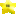Sprite DBBy Category
Welcome to the Sprite DB! Here you will find information on how to use any sprite in NSMB.
You can also have this database in NSMB Editor if you're using the latest version!

All the registered users can also collaborate with the Sprite DB by sharing their sprite data findings. Click any sprite below to edit it.

You need to be logged in to edit the sprite database.
All sprites

Unknown

Known, incomplete

Known, complete

Uncategorized
Platforms and ropes
Event controllers
Bosses and cutscenes
Camera controllers
Useless sprites
Effects and decorations
Other
Enemies - Overground
Enemies - Underground
Enemies - Water
Enemies - Snow
Enemies - Fire
Enemies - Castle
Enemies - Ghost House
Enemies - Desert

Go to sprite ID:
Status
326 total
292
32
2

The above bar needs more green. HELP US MAKE IT HAPPEN! NOW!
ID Class ID Name Last edited by
119 228Swinging Lift (Pendulum-like)
 Length: list at nybble 4: 0=4,2=8,3=12,4=16,5=20,6=24,7=28,8=32,9=36,10=40,11=44,12=48,13=52,14=56,15=60. Length formula is N4 × 4.1 acts like 0. Big sizes should not be used, as they don't display unless the pivot is on-screen. Amplitude: list at nybble 5: 0=0°,1=11.25°,2=22.5°,3=33.75°,4=45°,5=56.25°,6=67.5°,7=78.75°,8=90°,9=101.25°,10=112.5°,11=123.75°,12=135°,13=146.25°,14=157.5°,15=168.75° Oscillation period: list at nybble 6: 0=0 frame,1=1024 frames,2=512 frames,3=341.3 frames,4=256 frames,5=204.8 frames,6=170.7 frames,7=146.3 frames,8=128 frames,9=113.8 frames,10=102.4 frames,11=93.1 frames,12=85.3 frames,13=78.8 frames,14=73.1 frames,15=68.3 frames Starting position coefficient: value at nybble 7. For full details on the starting position formula, refer to this post: http://nsmbhd.net/post/43562/poudink (rev. 14)